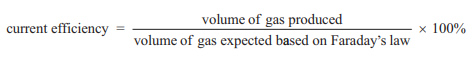Electrolysis (2019 VCE ) 1) A molten mixture of equal parts aluminium fluoride, AlF3, and sodium chloride, NaCl, undergoes electrolysis. Which one of the following statements about this reaction is correct? A. Sodium metal will be produced at the cathode and fluorine gas will be produced at the anode. B. Sodium metal will be produced at the anode and chlorine gas will be produced at the cathode. C. Aluminium metal will be produced at the cathode and chlorine gas will be produced at the anode. D. Aluminium metal will be produced at the anode and fluorine gas will be produced at the cathode Solution2) A student designed an experiment to investigate current efficiency during the electrolysis of a sodium chloride, NaCl, solution. Current efficiency is the amount of product produced, expressed as a percentage of the theoretical amount of product, calculated using Faraday’s law. When the products of an electrolysis are gases, current efficiency can be calculated using the following.a. Write the half-equation for the reaction that would be expected to be observed at the negative electrode. b. Calculate the volume of gas expected at the negative electrode for the experiment using Faraday’s law if a current of 2.00 amps was applied for 5.00 minutes. c.Calculate the current efficiency of the experiment. if 69.9 mL of gas was produced. Solution